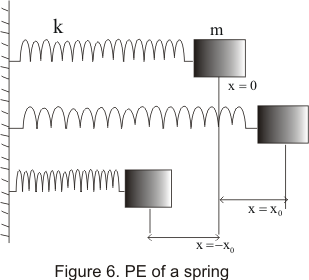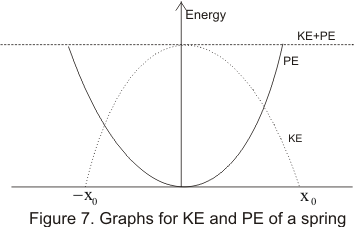# Potential energy of spring

## (9) Potential Energy of the spring

• To study this ,consider an electric spring of negligibly small mass .One end of the spring is attached to the rigid wall and another end of spring is attached to a block of mass m which can move on smooth frictionless horizontal surface
• Consider the figure given belowHere un-stretched or un-compressed position of the spring is taken at x=0
• We now take the block from its un-stretched position to a point P by stretching the spring
• At this point P restoring force is exerted by the spring on the block trying it bring it back to the equilibrium position.
• Similar restoring force developed in the spring when we try to compress it
• For an ideal spring ,this restoring force F is proportional to displacement x and direction of restoring force is opposite to that displacement
• Thus force and displacement are related as
F α x
or F= - kx           (16)
where K is called the spring constant and this equation (16) is known as Hook's law.negative sign indicates that force oppose the motion of the block along x
• To stretch a spring we need to apply the external force which should be equal in magnitude and opposite to the direction of the restoring force mentioned above i.e for stretching the spring
Fext=Kx Similarly for compressing the spring
- Fext= - Kx
or Fext=Kx (both F and x are being negative)
• Work done in both elongation and compression of spring is stored in the spring as its PE which can be easily calculated
• If the spring is stretched through a distance x from its equilibrium position x=0 then
W=∫Fextdx
Since both Fext and dx have same direction Now
W=∫Kxdx
On integrating with in the limits x=0 to x=x
We have
W=Kx2/2           (17)
• This work done is positive as force is towards the right and spring also moves towards the right
• Same amount of external is done on the spring when it is compressed through a distance x
• Work done as calculated in equation (17) is stored as Potential Energy of the spring.Therefore
U=Kx2/2           (18)

## (10)Conservation of energy of spring mass system

• Again consider the figure (6) where we have stretched the spring to a distance x0 from its equibrium position x=0
• If we release the block then speed of the block begin to increase from zero and reach its maximum value at x=0 (equilibrium position)
• Assuming that there is no dissipation of energy due to air resistance and frictional forces ,whole of the PE is converted in KE
• This means gain in KE is exactly equal to the loss in PE
• After reaching the equilibrium position at x=0 ,the block then crosses the equilibrium position ,it speed begin to decreases until it reaches the point x=-x0
• This would happen if whole the conversion between KE and PE is perfect without any dissipation effect
• Now at x=-x0 PE of the block is maximum ,KE is zero and the restoring force again pulls the block towards its equilibrium position.This way block would keep on oscillating
• From all discussion ,we see that total mechanical energy (KE+PE) of the system always remain constant which is equal to Kx02/2

• We can analyse this as follows

x=x0 : v=0 : KE=0 : PE=Kx02/2 : TE=Kx02/2
x=x: v=v : KE=mv2/2=k(x02-x2)/2 : PE=Kx2/2 : TE=Kx02/2
x=0 : v=vmax: KE=mvmax2/2=Kx02/2 : PE=0 : TE=Kx02/2
x=-x0: v=0 : KE=0 : PE=Kx02/2 : TE=Kx02/2

• Graph of KE and PE are shown below in figure• From above graph we can easily conclude that when KE increases PE decreases and when PE increase KE decrease but total energy of the system remains constant

• Above discussed case is the ideal case when other dissipated forces like frictional forces or air resistance are assumed to be absent
• However in reality some part of the energy of the system is dissipated due to these resistive forces and after some time system looses all its energy and come to rest